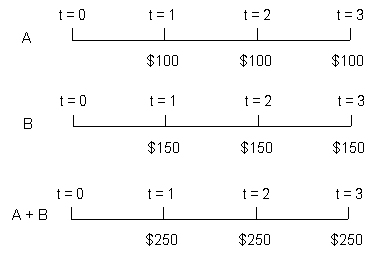### Seeing is believing!

Before you order, simply sign up for a free user account and in seconds you'll be experiencing the best in CFA exam preparation.

##### Subject 4. The Cash Flow Additivity Principle
The additivity principle: Dollar amounts indexed at the same point in time are additive.

Suppose we are considering two series of cash flows (A and B). The annual interest rate is 5%. We want to know the future value of combined cash flows at t = 3.• We can calculate the future value of each series and add them up. The future value of series A is 100 x 1.052 + 100 x 1.05 + 100 = 315.25 and the future value of series B is 150 x 1.052 + 150 x 1.05 + 150 = 472.875. The future value of A + B is 788.125.

• Alternatively, we can add the cash flows of each series first and then find the future value of the combined cash flows: 250 x 1.052 + 250 x 1.05 + 250 = 315.25 = 788.125.

We can use this principle to solve many uneven cash flow problems if we add dollars indexed at the same point in time. Consider a cash flow series, A, with \$100 indexed at t = 1, 2, 3 and 5, and \$0 at t = 4. This series is an almost-even cash flow, flawed only by the missing \$100 at t = 4. How do we find the present value of this series?

• We can create an annuity B with \$100 indexed at t = 1, 2, 3, 4, 5. It's easy to find the present value of this series.
• Then we isolate an easily evaluated cash flow B - A; it has a single cash flow of \$100 at t = 4. It's also easy to find the present value of this single cash flow.
• We then subtract the present value of B - A from the present value of B.

User Comment
BRENDAMBITHE YOUR study notes are simply the best although this is my first time I find them easy to understand
odette I really agree with you. I couldnt read QM from the text sent to me. But i am really enjoying reading it now.
TammTamm I understand this now but it would be nice to have a couple of basic questions.
lisalett I don't understand the lack of sample questions.
Metalpro I totally agree with BRENDAMBITHE. The notes will surely help me a lot.
vixignus yeah these are good
VikramJ we need questions!
TiredHand Analyst Notes describes it SO MUCH BETTER THAN THE CFA textbook.
MNSaleem I need help here, i am confused that through which formula The Future Value of Series A is determined, as t = 3 i expect calculation like 100 x 1.05(3) + 100 x 1.05(2) + 100.
OR also i think formula for Future value of regular annunity should be used.

So please some one make me clear. Thanks.
ybavly @MNSaleem

The diagram will make it clear. We are looking for the value AT t=3 not at the end of the year t=3.
This is still an ordinary annuity. The FV here happens on t=3 which is the first day of year 3. So, we simply add the \$100 because there was no time to reinvest it.
sgossett86 Using the BAII+ cf key makes solving uneven cash flows very simple.
Chl4072 Not understand at all

How to find it by using TI..?
saika Analyst Notes, you should create a tutorial for using cashflows. a small video for the calculator functions would help tremendously! Thanks.
thanks
Meruyet The additivity principle: Dollar amounts indexed at the same point in time are additive.
PLF1234 Good notes
Hi! Who knows how to use BA II for calculations?